# matplotlib.pyplot.subplot¶

`matplotlib.pyplot.``subplot`(*args, **kwargs)[source]

Add a subplot to the current figure.

Wrapper of `Figure.add_subplot` with a difference in behavior explained in the notes section.

Call signatures:

```subplot(nrows, ncols, index, **kwargs)
subplot(pos, **kwargs)
subplot(ax)
```
Parameters:
*args

Either a 3-digit integer or three separate integers describing the position of the subplot. If the three integers are nrows, ncols, and index in order, the subplot will take the index position on a grid with nrows rows and ncols columns. index starts at 1 in the upper left corner and increases to the right.

pos is a three digit integer, where the first digit is the number of rows, the second the number of columns, and the third the index of the subplot. i.e. fig.add_subplot(235) is the same as fig.add_subplot(2, 3, 5). Note that all integers must be less than 10 for this form to work.

projection : {None, 'aitoff', 'hammer', 'lambert', 'mollweide', 'polar', 'rectilinear', str}, optional

The projection type of the subplot (`Axes`). str is the name of a costum projection, see `projections`. The default None results in a 'rectilinear' projection.

polar : boolean, optional

If True, equivalent to projection='polar'.

sharex, sharey : `Axes`, optional

Share the x or y `axis` with sharex and/or sharey. The axis will have the same limits, ticks, and scale as the axis of the shared axes.

label : str

A label for the returned axes.

Returns:
axes : an `axes.SubplotBase` subclass of `Axes` (or a subclass of `Axes`)

The axes of the subplot. The returned axes base class depends on the projection used. It is `Axes` if rectilinear projection are used and `projections.polar.PolarAxes` if polar projection are used. The returned axes is then a subplot subclass of the base class.

Other Parameters:
**kwargs

This method also takes the keyword arguments for the returned axes base class. The keyword arguments for the rectilinear base class `Axes` can be found in the following table but there might also be other keyword arguments if another projection is used.

Property Description
`adjustable` {'box', 'datalim'}
`agg_filter` a filter function, which takes a (m, n, 3) float array and a dpi value, and returns a (m, n, 3) array
`alpha` float
`anchor` 2-tuple of floats or {'C', 'SW', 'S', 'SE', ...}
`animated` bool
`aspect` {'auto', 'equal'} or num
`autoscale_on` bool
`autoscalex_on` bool
`autoscaley_on` bool
`axes_locator` Callable[[Axes, Renderer], Bbox]
`axisbelow` bool or 'line'
`clip_box` `Bbox`
`clip_on` bool
`clip_path` [(`Path`, `Transform`) | `Patch` | None]
`contains` callable
`facecolor` color
`fc` color
`figure` `Figure`
`frame_on` bool
`gid` str
`in_layout` bool
`label` object
`navigate` bool
`navigate_mode` unknown
`path_effects` `AbstractPathEffect`
`picker` None or bool or float or callable
`position` [left, bottom, width, height] or `Bbox`
`rasterization_zorder` float or None
`rasterized` bool or None
`sketch_params` (scale: float, length: float, randomness: float)
`snap` bool or None
`title` str
`transform` `Transform`
`url` str
`visible` bool
`xbound` (lower: float, upper: float)
`xlabel` str
`xlim` (left: float, right: float)
`xmargin` float greater than -0.5
`xscale` {"linear", "log", "symlog", "logit", ...}
`xticklabels` List[str]
`xticks` list
`ybound` (lower: float, upper: float)
`ylabel` str
`ylim` (bottom: float, top: float)
`ymargin` float greater than -0.5
`yscale` {"linear", "log", "symlog", "logit", ...}
`yticklabels` List[str]
`yticks` list
`zorder` float

Notes

Creating a subplot will delete any pre-existing subplot that overlaps with it beyond sharing a boundary:

```import matplotlib.pyplot as plt
# plot a line, implicitly creating a subplot(111)
plt.plot([1,2,3])
# now create a subplot which represents the top plot of a grid
# with 2 rows and 1 column. Since this subplot will overlap the
# first, the plot (and its axes) previously created, will be removed
plt.subplot(211)
```

If you do not want this behavior, use the `Figure.add_subplot` method or the `pyplot.axes` function instead.

If the figure already has a subplot with key (args, kwargs) then it will simply make that subplot current and return it. This behavior is deprecated. Meanwhile, if you do not want this behavior (i.e., you want to force the creation of a new suplot), you must use a unique set of args and kwargs. The axes label attribute has been exposed for this purpose: if you want two subplots that are otherwise identical to be added to the figure, make sure you give them unique labels.

In rare circumstances, `add_subplot` may be called with a single argument, a subplot axes instance already created in the present figure but not in the figure's list of axes.

Examples

```plt.subplot(221)

# equivalent but more general
ax1=plt.subplot(2, 2, 1)

# add a subplot with no frame
ax2=plt.subplot(222, frameon=False)

plt.subplot(223, projection='polar')

# add a red subplot that shares the x-axis with ax1
plt.subplot(224, sharex=ax1, facecolor='red')

#delete ax2 from the figure
plt.delaxes(ax2)

#add ax2 to the figure again
plt.subplot(ax2)
```

## Examples using `matplotlib.pyplot.subplot`¶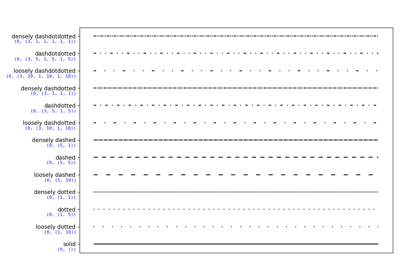Linestyles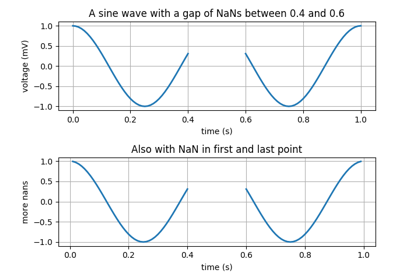Nan Test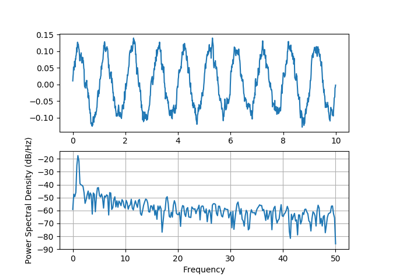Psd Demo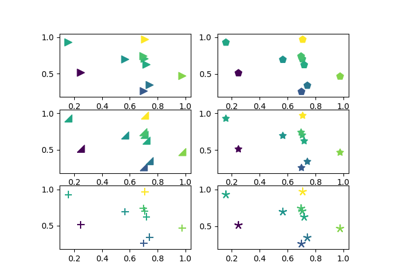Scatter Star Poly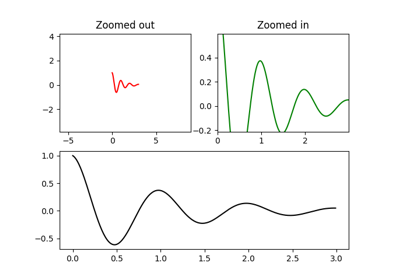Zooming in and out using Axes.margins and the subject of "stickiness"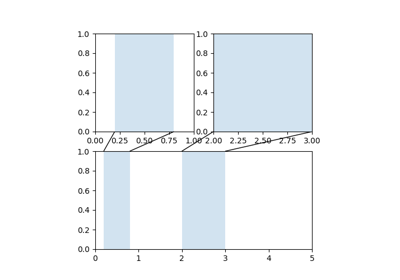Axes Zoom Effect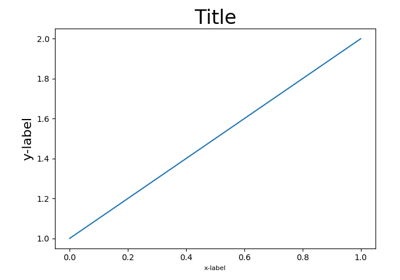Resizing axes with tight layout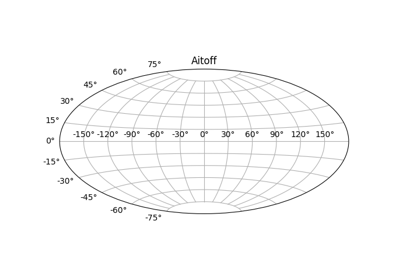Geographic Projections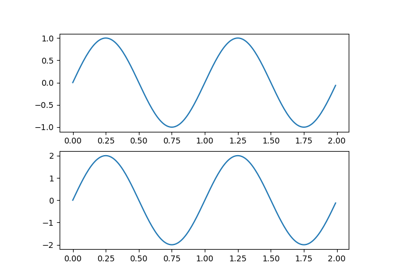Multiple Figs Demo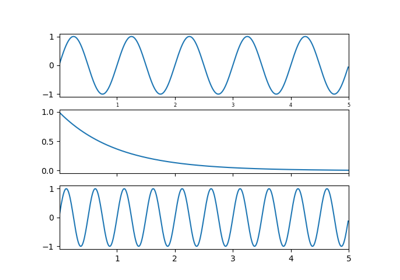Shared Axis Demo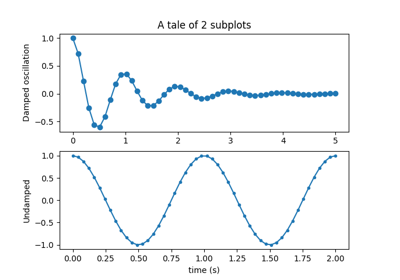Multiple subplots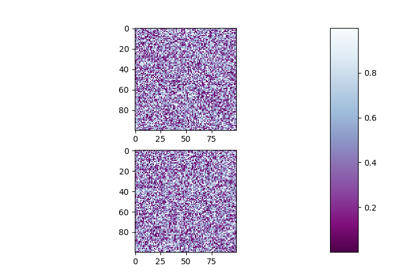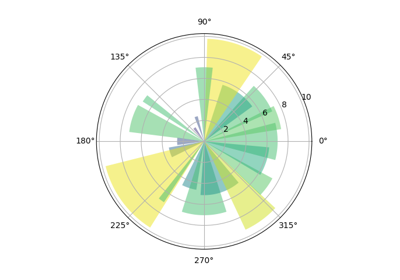Pie chart on polar axis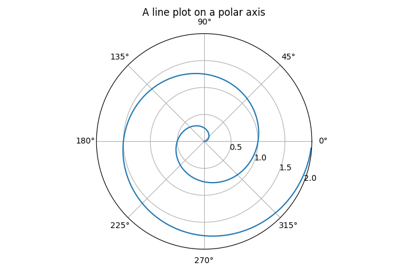Polar Demo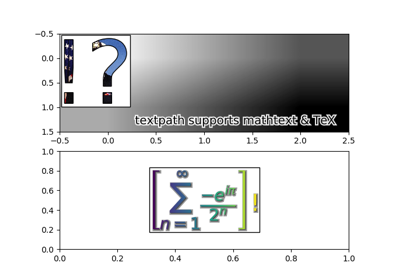Demo Text Path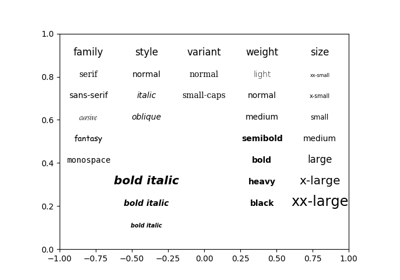Fonts demo (object-oriented style)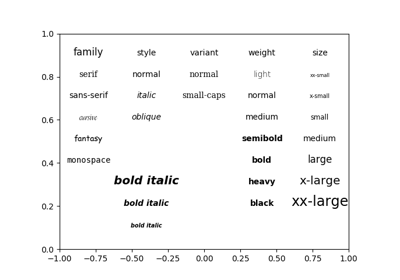Fonts demo (kwargs)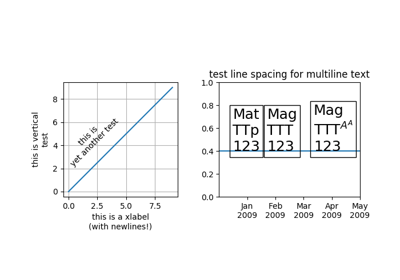Multiline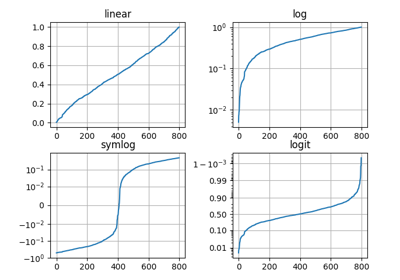Pyplot Scales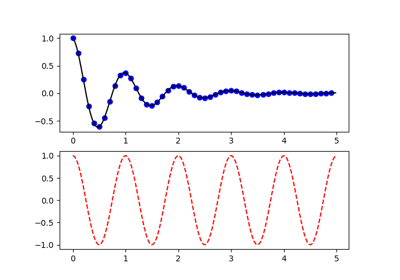Pyplot Two Subplots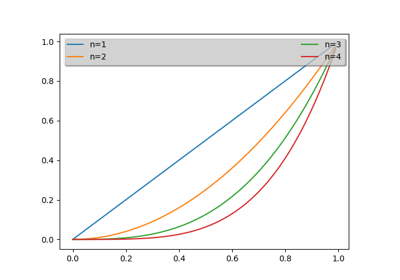Whats New 0.98.4 Legend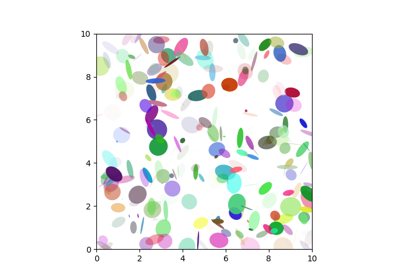Ellipse Demo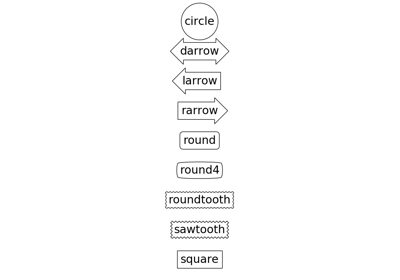Fancybox Demo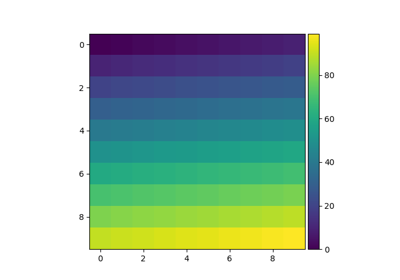Simple Colorbar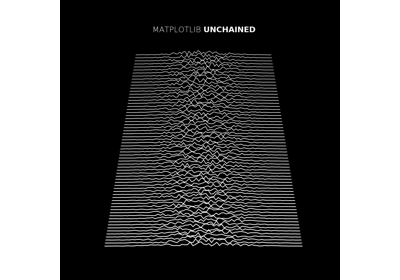MATPLOTLIB UNCHAINED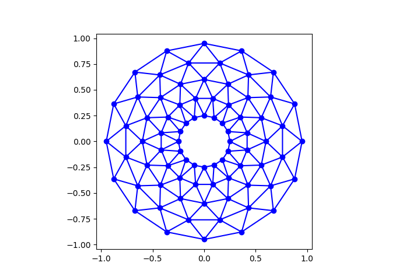Trifinder Event Demo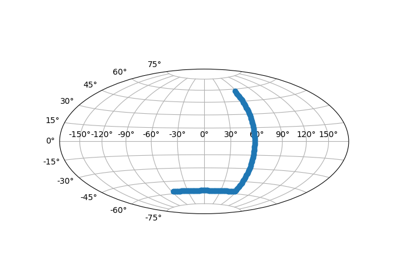Custom projectionCustomize Rc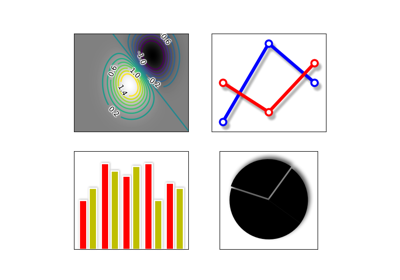Demo Agg Filter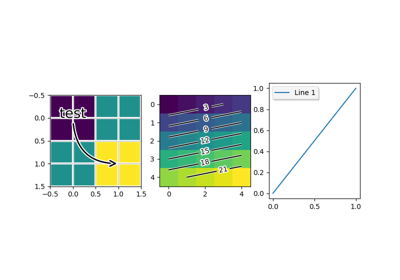Patheffect Demo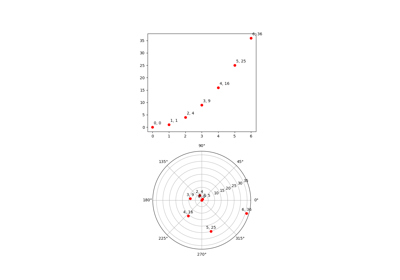Transoffset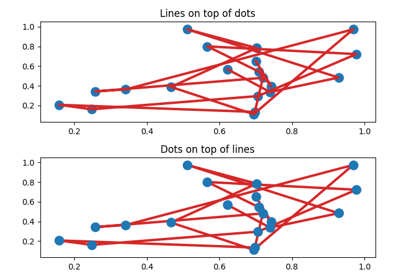Zorder Demo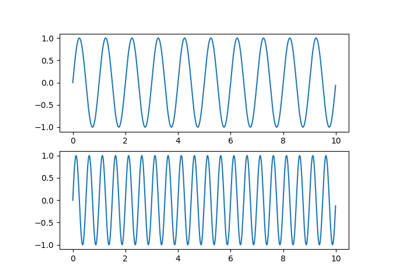Sharing axis limits and views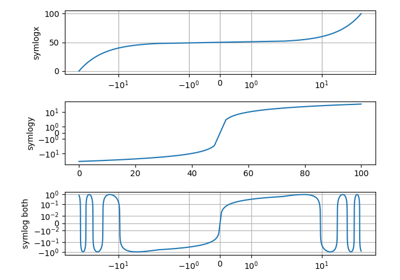Symlog Demo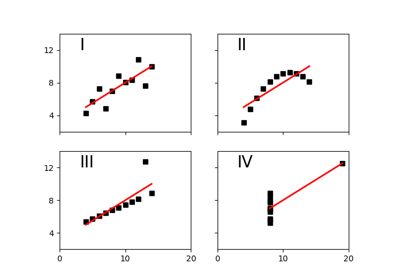Anscombe's Quartet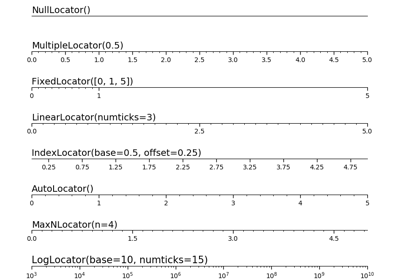Tick locators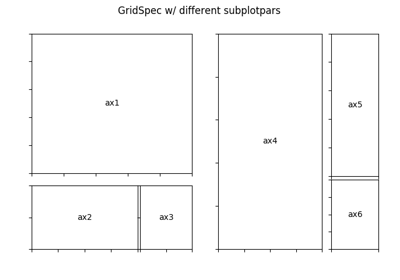Demo Gridspec03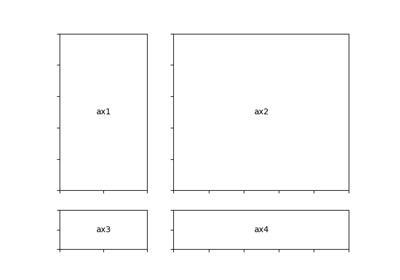Demo Gridspec05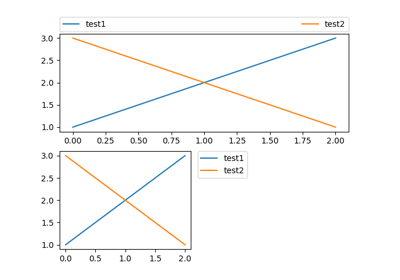Simple Legend01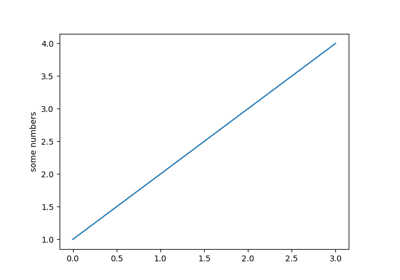Pyplot tutorial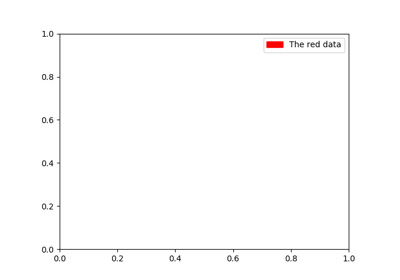Legend guide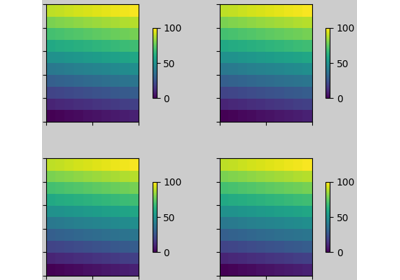Constrained Layout Guide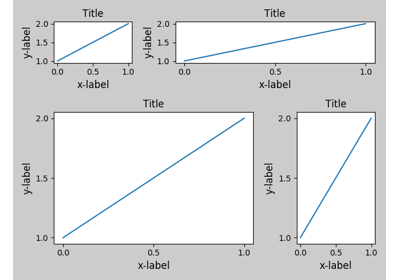Tight Layout guide